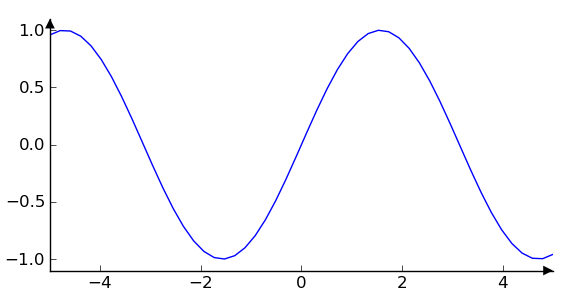# Is it possible to plot axes with arrows ?

Hello,

I use matplolib by the mathematical system Sage in order to plot a function.
The Sage code calls matplotlib and uses its options : The Sage command is

plot (sin, x, -5, 5)

I add labels par axes_labels or remove axes by :

plot (sin(x), x, -5, 5, axes_label = ['x', 'y'])
plot (sin(x), x, -5, 5, axes=false)

French users (and maybe others) uses arrows and not lines for axes.
I'm looking for a plot (sin(x), x, -5, 5, axes="arrows")
Is there a pretty way to get these arrows. The result of this code isn't so fine.
length, width and color don't match.

plot (sin(x), x, -5, 5, axes=false) + arrow ((-5,0),(5,0)) + arrow ((0,-1),(0,1))

What options do you propose ?
I don't find relevant answers in the archive.

F. from France.

Cc:
cc:
X-PGP-Key: http://pirsquared.org/PaulIvanov0F3E28F7.asc

Francois Maltey, on 2011-02-02 15:51, wrote:

Hello,

I use matplolib by the mathematical system Sage in order to plot a function.
The Sage code calls matplotlib and uses its options : The Sage command is

plot (sin, x, -5, 5)

I add labels par axes_labels or remove axes by :

plot (sin(x), x, -5, 5, axes_label = ['x', 'y'])
plot (sin(x), x, -5, 5, axes=false)

French users (and maybe others) uses arrows and not lines for axes.
I'm looking for a plot (sin(x), x, -5, 5, axes="arrows")
Is there a pretty way to get these arrows. The result of this code isn't
so fine.
length, width and color don't match.

plot (sin(x), x, -5, 5, axes=false) + arrow ((-5,0),(5,0)) + arrow
((0,-1),(0,1))

What options do you propose ?
I don't find relevant answers in the archive.

Hi Francois,

I'm not sure I understand - but do you want the arrows at the end
of the axes spines? I don't think there's a direct way to adjust
the spines to become arrows at the moment, but we can remedy that
by making annotations in axes coordinates. The important thing
to know here is that in axes coordinates, which are always
between 0-1, spine endpoints are at these locations: (0,0),
(0,1), (1,0), and (1,1). Here's the code, and attached is the
resulting image

import matplotlib.pyplot as plt
ax = plt.subplot(1,1,1)

al = 7 # arrow length in points
arrowprops=dict(clip_on=False, # plotting outside axes on purpose
frac=1., # make end arrowhead the whole size of arrow
facecolor='k')
kwargs = dict(
xycoords='axes fraction',
textcoords='offset points',
arrowprops= arrowprops,
)

ax.annotate("",(1,0),xytext=(-al,0), **kwargs) # bottom spine arrow
ax.annotate("",(0,1),xytext=(0,-al), **kwargs) # left spin arrow

# hide the top and right spines
[sp.set_visible(False) for sp in ax.spines['top'],ax.spines['right']]

#hide the right and top tick marks
ax.yaxis.tick_left()
ax.xaxis.tick_bottom()

x = np.linspace(-5,5,50)
ax.plot(x, np.sin(x))

# adjust the view a little bit
ax.set_xlim(-5,5)
ax.set_ylim(-1.1,1.1)
plt.draw()

I'm not familiar with how SAGE exposes matplotlib functionality,
though, since the syntax you used differs from how matplotlib is
utilized.

best,···

From: Paul Ivanov <pivanov314@...287...>
To: Francois Maltey <fmaltey@...3425...>
Subject: Re: [Matplotlib-users] Is it possible to plot axes with arrows ?
--
Paul Ivanov
314 address only used for lists, off-list direct email at:
http://pirsquared.org | GPG/PGP key id: 0x0F3E28F7

I've made a Sage ticket for this:

http://trac.sagemath.org/sage_trac/ticket/10740

As a clumsy workaround, you could use the .matplotlib() method for Sage graphics objects to get the matplotlib figure object for the graphics object. Then you could:

1. figure out which spine was being used as the drawn axes
2. use that spine's transform to place an arrow at the end of the spine (using the example code that was just posted in another message to draw the arrow).
3. Draw the figure

Thanks,

Jason

···

On 2/2/11 8:51 AM, Francois Maltey wrote:

Hello,

I use matplolib by the mathematical system Sage in order to plot a function.
The Sage code calls matplotlib and uses its options : The Sage command is

plot (sin, x, -5, 5)

I add labels par axes_labels or remove axes by :

plot (sin(x), x, -5, 5, axes_label = ['x', 'y'])
plot (sin(x), x, -5, 5, axes=false)

French users (and maybe others) uses arrows and not lines for axes.
I'm looking for a plot (sin(x), x, -5, 5, axes="arrows")
Is there a pretty way to get these arrows. The result of this code isn't
so fine.
length, width and color don't match.

plot (sin(x), x, -5, 5, axes=false) + arrow ((-5,0),(5,0)) + arrow
((0,-1),(0,1))

What options do you propose ?